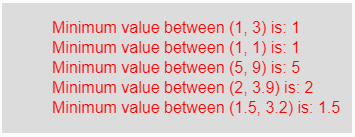# p5.js | min() function

• Last Updated : 09 Apr, 2019

The min() function in p5.js is used to get the minimum value from the sequence of numbers.

Syntax:

`min(a, b)`

or

`min(arr)`

Parameters: The min(a, b) function accepts two parameters which are two different number and compared to get minimum value among them. The min(arr) function accepts a single parameter array.

Return Value: It returns the minimum value among the different numbers.

Below program illustrates the min() function in p5.js:

Example: This example uses min() function to get the minimum value.

 `function` `setup() {  ` `  `  `    ``// Create Canvas of size 270*80  ` `    ``createCanvas(350, 130);  ` `}  ` `  `  `function` `draw() {  ` `      `  `    ``// Set the background color  ` `    ``background(220);  ` `      `  `    ``// Call to min() function  ` `    ``let u = min(1, 3); ` `    ``let v = min(1, 1); ` `    ``let w = min(5, 9); ` `    ``let x = min(2, 3.9); ` `    ``let y = min(1.5, 3.2); ` `      `  `    ``// Set the size of text  ` `    ``textSize(16);  ` `      `  `    ``// Set the text color  ` `    ``fill(color(``'red'``));  ` `    `  `    ``// Getting minimum value     ` `    ``text(``"Minimum value between (1, 3) is: "` `+ u, 50, 30); ` `    ``text(``"Minimum value between (1, 1) is: "` `+ v, 50, 50); ` `    ``text(``"Minimum value between (5, 9) is: "` `+ w, 50, 70); ` `    ``text(``"Minimum value between (2, 3.9) is: "` `+ x, 50, 90); ` `    ``text(``"Minimum value between (1.5, 3.2) is: "` `+ y, 50, 110);      ` `}  `

Output:Reference: https://p5js.org/reference/#/p5/min

My Personal Notes arrow_drop_up
Recommended Articles
Page :